Courses

# Ex 7.1 NCERT Solutions- Coordinate Geometry Class 10 Notes | EduRev

## Class 10 : Ex 7.1 NCERT Solutions- Coordinate Geometry Class 10 Notes | EduRev

The document Ex 7.1 NCERT Solutions- Coordinate Geometry Class 10 Notes | EduRev is a part of the Class 10 Course Mathematics (Maths) Class 10.
All you need of Class 10 at this link: Class 10

Q.1. Find the distance between the following pairs of points:
(i) (2, 3), (4, 1)
(ii) (-5, 7), (-1, 3)
(iii) (a, b), (-a, -b)
Sol. (i) Let A(2, 3) and B(4, 1)
The distance between two points is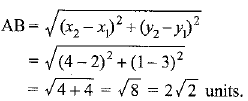(ii) Let A (-5, 7) and B (-1, 3)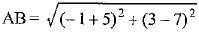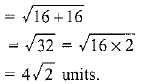(iii) Let A (a, b) and B (-a, -b)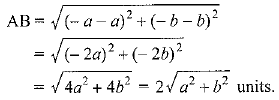Q.2. Find the distance between the points (0, 0) and (36, 15). Can you now find the distance between the two towns A and B discussed in Section 7.2?
Sol. Let points be A (0, 0) and B (36, 15)
The distance between two points is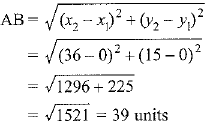Q.3. Determine if the points (1, 5), (2, 3) and (-2, -11) are collinear.
Sol. Let points be A(1, 5), B(2, 3) and C(-2, -11)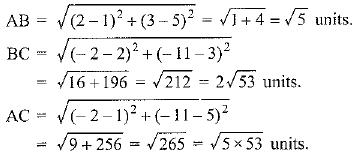AB + BC ≠ AC
Hence, the given points are not collinear.

Q.4. Check whether (5, -2), (6, 4) and (7, -2) are the vertices of an isosceles triangle.
Sol. Let the points be A (5, -2), B (6, 4) and C (7, -2).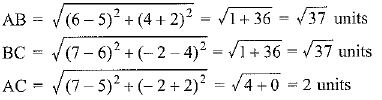Here AB = BC
∴ Δ ABC is an isosceles.

Q.5. In a classroom, 4 friends are seated at the points A, B, C and D as shown in Fig. Champa and Chameli walk into the class and after observing for a few minutes Champa asks Chameli, ‘‘Don’t you think ABCD is a square?’’ Chameli disagrees. Using distance single formula, find which of them is correct.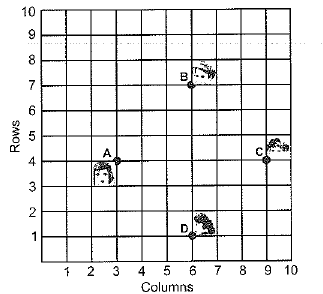Sol. Points A (3, 4), B (6, 7), C (9, 4) and D (6, 1)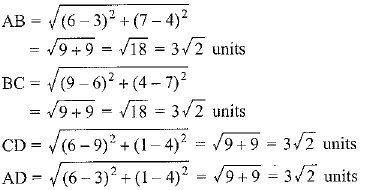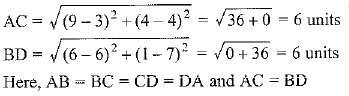∴ ABCD is a square.
Hence, Champa is correct.

Q.6. Name the type of quadrilateral formed, if any, by the following points, and give reasons for your answer:
(i) (-1, -2), (1, 0), (-1, 2), (-3, 0)
(ii) (-3, 5), (3, 1), (0, 3), (-1, - 4)
(iii) (4, 5), (7, 6), (4, 3), (1, 2)
Sol. (i) Let the points be: A (-1, -2), B (1, 0), C (-1, 2) and D (-3, 0).
The distance between two points is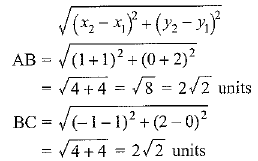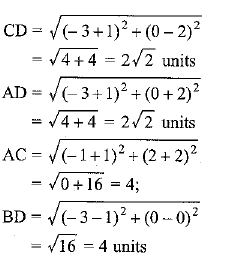Here AC - BD, AB = BC = CD = AD
Hence, the quadrilateral ABCD is a square.
(ii) Let points be A (-3, 5), B (3, 1), C (0, 3) and D (-1, -4)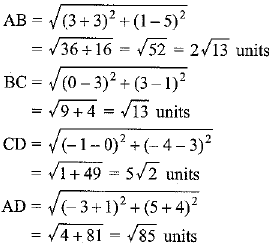The given points do not form any quadrilateral.
(iii) Let points be A (4, 5), B (7, 6), C (4, 3) and D (1, 2)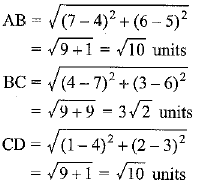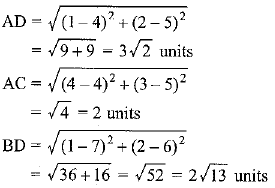Here, AB = CD, BC = AD and AC ≠ BD
∴ The quadrilateral ABCD is a parallelogram.

Q.7. Find the point on the x-axis which is equidistant from (2, -5) and (-2, 9).
Sol. Let points be A (2, -5) and B (-2, 9)
Let P (x, 0) be the point on x-axis.
∴ PA = PB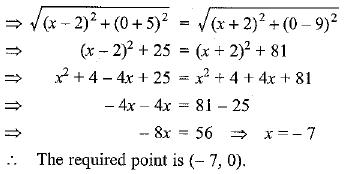Q.8. Find the values of y for which the distance between the points P(2, -3) and Q(10, y) is 10 units.
Sol. Points P (2, -3), Q (10, y) and PQ = 10 units
The distance between two points is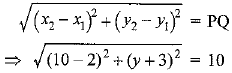⇒ y - 3 = 0 or y + 9 = 0
⇒ y = 3 or - 9

Q.9. If Q (0, 1) is equidistant from P (5, -3) and R (x, 6), find the values of x. Also, find the distances QR and PR.
Sol. PQ = QR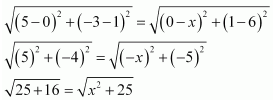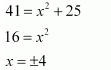Therefore, point R is (4, 6) or (−4, 6).
When point R is (4, 6),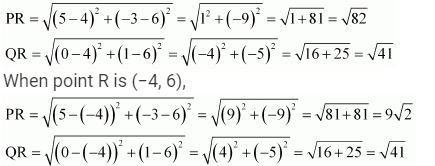Q.10. Find a relation between x and y such that the point (x, y) is equidistant from the point (3, 6) and (-3, 4).
Sol. Points A (3 , 6), B (-3, 4) and point P(x, y) is equidistant from points A and B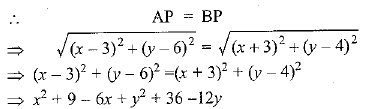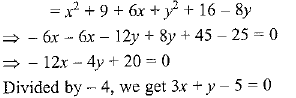Offer running on EduRev: Apply code STAYHOME200 to get INR 200 off on our premium plan EduRev Infinity!

## Mathematics (Maths) Class 10

178 videos|268 docs|103 tests

,

,

,

,

,

,

,

,

,

,

,

,

,

,

,

,

,

,

,

,

,

;